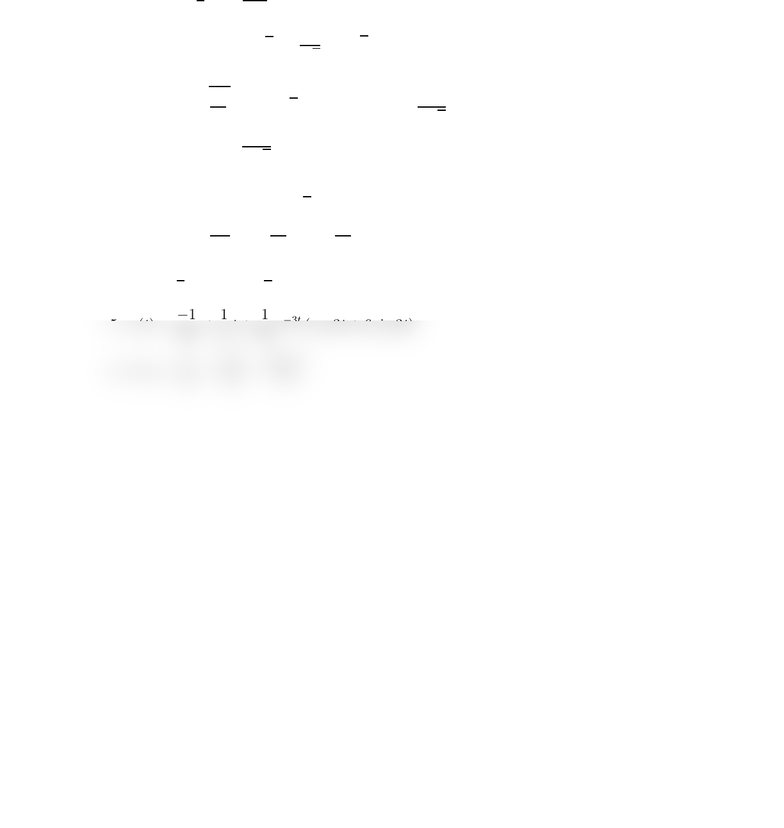# CHEM 110 NIU CHEM110 Practice Exam 3 Key

22 views1 pages
School
Course
ProfessorMATH 336–4 Answers to Exam 3 Fall 2015
Prof. Ammar
1. yp(x) = 1
4x4+ln x
2x4
2. (a) x(t) = 3 cos 3t+1
3sin 3t, assuming the positive direction is to the right, and
the negative of this if the positive direction is to the left.
(b) C=s28
3,ω0=3, α=π+ tan1 1
33!2.9515 if positive is to the right, or
α= tan1 1
33!≈ −0.1901 if positive is to the left.
3. (a) f(t) = e3t3 cos 4t+5
2sin 4t
(b) f(t) = 1
25 e2t+1
25 e3t+11
5te3t
4. x(t) = 1
2et+e4t1
2e3t
5. x(t) = 1
18 +1
6t+1
18 e3t(cos 3t+ 6 sin 3t)
6. F(s) = 1
s2e2s
s22e4s
s
Unlock document

This preview shows half of the first page of the document.
Unlock all 1 pages and 3 million more documents.